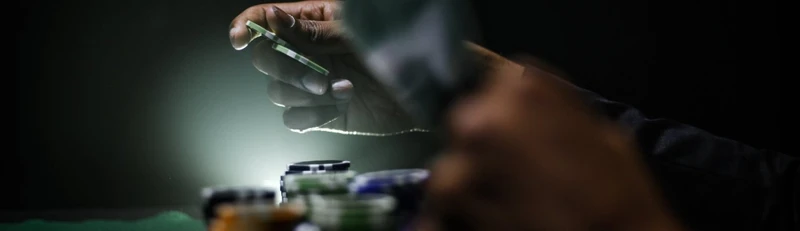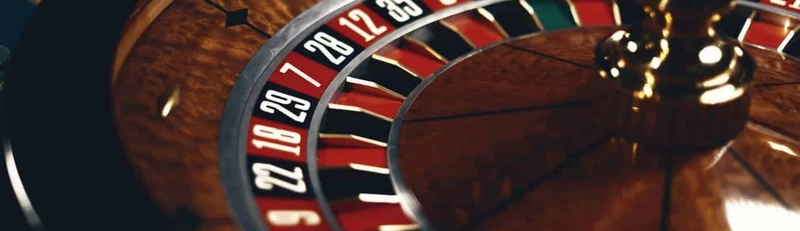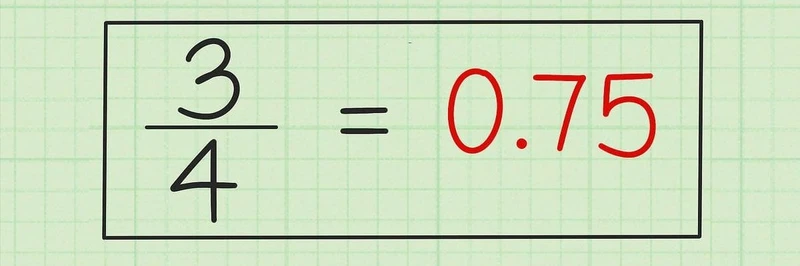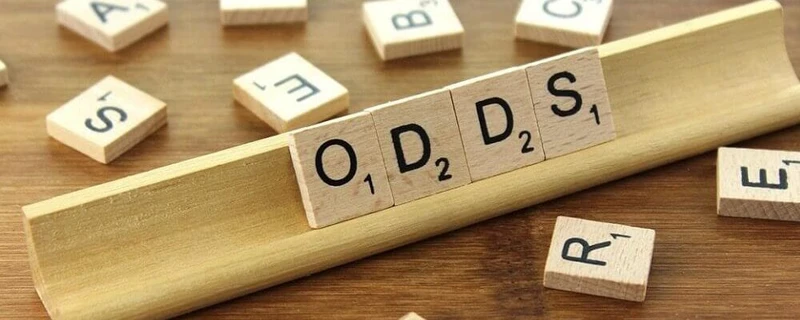# How To Understand Betting Odds: Fractions vs DecimalsWritten by Phoebe

Published date · Nov. 27, 2020 | Last Updated · July 4, 2022 | Read Time: 4 minsDo you have a good idea of how to read odds when you’re placing a bet? Do you know how to go from fractions to decimals? Understanding betting odds and odds conversion is essential if you want to enjoy gambling to the fullest. After all, don’t you want to know how much you stand to win when you log on to a betting site and place a bet? It can seem a little tricky at first, especially if you never paid much attention during maths class in school. However, if you keep reading, you’ll find that things aren’t as complicated as you first thought.## Probability Within Casino Games

First and foremost, the most important concept behind betting odds is probability. This is the chance, or likelihood, of an event happening. When it comes to a coin toss, calculating the probability is simple: There’s a 50% chance of tails and a 50% chance of heads. Games like roulette and card games also have clear-cut probabilities. However, when it comes to sporting events, the probability depends on millions of different factors. Here, the “experts” have to guess the probability using expertise and judgement. For example, if Manchester United are on top form and top of the league then the probability of them beating the bottom of the league team is pretty high.

With casino games, there are clear-cut probabilities and, therefore, odds can be calculated precisely.  In European roulette, you have 37 numbers on the wheel (including the green zero). Imagine you bet £1 on a number. When the wheel is spun you have a 2.7% chance of winning. (We’ll come to calculations in a short while). What’s important to understand is that your payout, upon winning, is £35. However, you will get £36 back including the £1 that you bet.## Fractions vs Decimals

Odds are usually presented in two ways: fractions or decimals. With fractions, you will see one number (the numerator), a forward slash and a second number (the denominator). If you toss a coin, the odds would be expressed as 1/1 (in this case both numerator and denominator are 1).

If the first number is bigger than the smaller then it’s considered to be odds against. The other way around and it’s odds on. Odds on means the event is more likely to happen than not.

Let’s say you see the odds: 100/1. If you bet £1 then you’ll receive £101 back, consisting of £100 winnings, plus your stake of £1.

How do you go from 1/1 to 50% in the case of a coin toss? If you represent the fraction odds as X/Y, the formula would be:

Percentage = Y / (X + Y) x 100

So, 1 / (1 + 1) x 100 = 50%

You can apply this formula whenever you want to convert fractional odds to a percentage and vice versa. In the case of betting on a single number in roulette:

The odds as a fraction are 36/1. To get the percentage:

1 / (36 + 1) * 100 = 2.7%

This means you have a 2.7% (or a one in 37) chance of winning.

It’s important to recognise that the payout doesn’t match the chance of winning. You may be wondering, if there are 37 numbers, why do you only receive £36 and not £37 upon winning? This small difference is known as the house edge and is what allows casinos to make a small profit.## Converting Fractions to Decimals

When it comes to decimal odds, the number you see includes the stake. For odds of 15.00, you would receive a total of £15 if you placed a winning bet of £1.

How do you convert to decimal odds? To go from fractional odds to decimal, use the following formula:

Decimal odds = X + Y

So, if you see odds of 10/1, decimal odds = (10 + 1) = 11.00

Remember that a decimal is simply a percentage divided by 100. The percentage is referred to as implied probability and to go from decimal odds to percentage, you simply use the formula:

(1/decimal odds) x 100 = Implied probability## The American Way

As if things weren’t complicated enough, there is another method to understand as you’re on your way to becoming an expert odds converter. The Americans will display their odds as a number with a plus or minus in front of it. It’s quite straightforward to understand and convert American odds though.

Let’s say there’s an American football game this afternoon. You may see the following odds:

New York Giants @ -200

Dallas Cowboys @ +300

If you were to bet £100 on the Dallas Cowboys and they win, you would receive £400 (£300 winnings plus your stake of £100).

Since the New York Giants have a negative number, they are favourites and the calculation is a little different. Here, you simply win £100 for every £200 that you bet.

To convert American odds to decimal for a favourite, in the case of the New York Giants, it would be 1.50. For the Dallas Cowboys, a the underdogs, the odds conversion would be 4.00.

## In Summary

Most online casinos tend to calculate and display their odds as fractions. However, if you dislike fractions then you’re in luck. Casino sites give you an option to display odds as fractions or decimals. This means you don’t have to become a master of mathematics and perform calculations each time you place a bet. Phew!

If you’ve enjoyed doing some maths and like the process of working out odds then great! If not, then don’t worry. As long as you understand the underlying concept of probability, you’ll be fine. Just remember, whether it’s decimals, fractions, percentages, or the American way, all you’re working out is the likelihood of an event occurring… and more importantly, of course, how much you stand to win!

## Top Recommended Casino To Play Online Casinos Explained